main page   list of papers   library

AETHEREAL INERTIA
Dedicated to he bright memory of my daughter Anastasia

Abstract. The fallacies of the modern mechanics which has arisen still in epoch of Isaac Newton are considered. The concept and origin of inertia as attribute of aether is given. The gear of inertial properties of aether is uncovered. It is shown that Galilean laws of inertia and Galilean principle of relativity are effects of properties of aether.

Reasons of fallacies in mechanics

Paradoxical and illogical concepts of modern physics concerning mechanical characteristics of substance and field, there were not today and not hundred years ago. For comprehension of true situation it is necessary to return to 17th century, when Isaac Newton has proclaimed as own two Galilean laws of mechanics and one law of Huygens, and the political forces have proclaimed Newton as “almost divine mind”. All would be tolerant, if alchemist and manager of British Royal Mint Isaac Newton, having borrowed these laws, would not make a “hypothesis fingo”.

Galilean Law of Inertial Motion

The deeply physical Galilean Law of Inertia , states (in the modern formulation):

Any physical body, still or moving in a physical environment with constant speed is rectilinear or rotating around of a centre of mass, will prolong this motion eternally if other physical bodies or environment will not exert resistance to this motion. Such motion is inertial motion”.

Isaac Newton has transformed this law in the formulation, 1687 :

“Corpus omne perseverare in statu suo quiescendi vel movendi uniformiter in directum, nisi quatenus illud a viribus impressis cogitur statum suum mutare”

“Every body is in state of rest or uniform rectilinear motion unless an external force is applied to it”

In the modern formulation so-called “the First Newtonian Law”  sounds worse:

“Every mass point saves a state of rest or uniform rectilinear motion unless an external force is applied to it”

Thus the pure experimental physical law discovered by Galileo in 1612 - 1638, sharpened in 1644 by Rene Descartes  and by Christian Huygens, and widely known by the time when Isaac Newton leaved alone his alchemy and began physical and mathematical activity. Newton has transformed that law to a philosophical nonsense – the motion of an abstract “material point” in vacuum. Three rotary degrees of freedom were excluded and the environment - carrier of physical motion were eliminated.

I perceive how it is difficult for modern man for whom the motion in vacuum was inserted into a mind at a level of the instinct and dogmatic faith, to realize illogicalness of motion in absolute emptiness, the mismatch of Newtonian interpretation to realities of Nature. However, not losing hope for comprehension, I will try to present my point of view to the reader.

If the motion of any physical system happened in absolute (abstract) vacuum, it would be impossible to distinguish even logically this motion from the rest, as the vacuum has no distinctive tags (labels), on which it would be possible to detect this motion. Given “mathematical property” is utilized as the substantiation of relativism, though this “property” exists only in the theory, in minds of relativists, but not in Nature.

Here it is necessary to mark, that the phenomenological relativity of Galileo, if we do not accent the attention on the trivial mathematical side - Cartesian transformation of coordinates, approves only that at usual low velocities, with which the people face in daily life, the difference between inertial frames is not felt. For aethereal environment these velocities are so insignificant that the physical phenomena flow equally.

On the other hand, the linear motion measured in vacuum concerning other bodies, can not be an objective unambiguous measure of motion, as it depends on arbitrariness of the observer that is selection of a reference frame. In the terms of linear motion it is possible to consider velocity of a stone lying on ground equal to zero if we take Earth as a reference frame, and equal 30 km/s if we take Sun as a reference frame.

The rotary movement, declared as a particular case and which has been thrown out by Newton from the formulation of the Law of Inertia as against a headway, is absolute and unambiguous, as certainly the Universe is not spun around of any stone.

Thus, originally pure phenomenological Galilean Law was cut off on three degree of freedom, is deprived of the physical environment and is converted into a certain abstract dogma stopped development of mechanics and physics in whole, having closed thoughts of physicists only in linear relative motion.

Galilean Law on Inertial Force

Galilean Law discovered four centuries ago  states in the modern formulation:

“Change of momentum of a body to the force, equally applied(put) on it(him), change of a moment of momentum of a body to the equally applied(put) moment of forces ”.

As a matter of fact it is the formulation of a conservation law of momentum and energy, that is statement(confirmation) of a causality of a mechanics.

The modern formulation of this law about same, truth, he pirately is named “ as the second Newton's law ”.

Newton reformulated this law of Galilei in own way :

“Mutationem motus proportionalem esse vi motrici impressae et fieri secundum lineam rectam qua vis illa imprimitur”

“The rate of change of momentum of is proportional to the resultant force acting on the body and is in the same direction”

And

“The acceleration of material point directly proportional to a force, operational on it, and in inverse proportion to its mass”

Not having understood neither generality of the Galilean formulation, nor its true physical sense, Newton has bended consciousness of succeeding generations of physicists, having suppressed their thinking by force of politically acquired authority.

Let's consider these distortions.

Five distortions of mechanics

The first distortion concerns rotary movement, which Newton has found as a particular case of rectilinear motion. However, it is not so. The rotary motion, as against rectilinear motion, is absolute, and will be subordinated absolutely to other laws, it responses to the physical environment in other manner. It is easy for seeing on an example of the gyro (fast rotated body) which has other inertial properties rather than rectilinearly moving body. Inertia, which is a causal response delay, is a response to force in a case of rectilinear motion, and in case of rotary motion it is a response to the moment of forces.

The second distortion consists in Newton’s accepting of a concept of “absolute vacuum”, emptiness of Space. If we accept that the inertial forces arise from nowhere, it is necessary to recognize it “divine”, as it was done by Newton, and accept physics as a section of theology. If we recognize these forces fictitious, as it is done by modern peripatetics (for example see [5,6]), it is necessary to refuse the principle of causality in physics, that is again we must exclude physics from science. Recognition of reality of inertial forces means the recognition of existence of environment, carrier of those forces that is aether.

The third distortion consists in identifying of inertia and weight. If for Galileo the inertia of weighable bodies was proportional to weight, that corresponds to a physical reality, for Newton it is equivalents, that is not the same and it does not correspond to true, as, for example, the photon, having inertia (momentum and moment of momentum) has no gravitational mass. Same it is possible to say about Einstein’s “principle of equivalence”, which does not confirm by practice.

The fourth distortion consists in statement about centrality of gravitational force, outgoes from wrong Newton’s understanding of Galilean Law of Inertia and "modification” of Hook’s Law of Gravitation, from which Newton has thrown out aether. Isaac Newton has accepted the centripetal force for the basis, which actually is response to the natural inertial force only. This fallacy is refuted by modern experiments indicating that the force of gravitation differs from central. Theoretically the theory of Newton is refuted by development of ideas of Hooke - Bernoulli - Euler - Gauss – Gerber the offered by the author [7, 8].

The listed above and mathematical difficulties of the description of gravitation in the terms of short-range interaction and continuous energy transmission in environment, have resulted in the fifth Newton’s distortion – declaring a postulate of instantaneous remote action, violation of a principle of a causality, general for all sciences.

The distortions, brought by Newton in concepts of inertia, mass and gravitation are living till now. They hinder to development of physics and new technologies, hiding a true Nature from a human eyes and mind. Therefore we will consider, of what they consist.

As it was shown by the author earlier , the conceptual chaos reigns in a modern physics, in which the concepts of mass, inertia and gravitation are mixed. As for comprehension of further presentation it is important to understand it let’s try to consider it in detail.

The Concept of Mass

The mass is a measure of quantity of substance.

As well as any other measures, the measure of mass should have properties, mandatory for patrimonial concept, the measure. Mandatory, attributive property of any measure the additivity is. It is bound rigidly with concept of measurability . In other case that is not a measure. The property of additivity and independence from a way of measurement makes one a measure. Therefore talks about mass varying depending on an aspect angle of its observation transform physics in circus booth.

It is possible to point following basic ways of measurement of mass:

- Scaled, concluding in count of number of identical mass units (particles, atoms, molecules…);

- Volumetric, concluding in measurement of a volume of material of identical density (counters of liquids, measurement volumes);

- Gravitational, concluding in sensing of the gravitational force made by interaction of given mass with gravitational field (balance and static spring balance);

- Inertial, concluding in measurement of inertial forces arising at metrology motion of mass (dynamic spring balance, vibrators).

The Concept of Inertia

Modern physicists immix concept of inertia with concept of mass. This mistake originates from Newton’s transactions, who has introduced the postulate of absolute empty Space, has eliminated aether, having made inertial forces fictituous, arising as “divine” attribute of mass.

Actually inertia, as it was shown by Nikolay Umov [11,12], is an effect of a causality, continuity of process of transmission of momentum and energy in a material medium without dependence from a concrete nature of a material. Umov’s conclusive rule about continuity of energy, that the energy is propagate not only in definite space, but also in definite period, eliminates any thought about Newtonian instantaneous long-range action .

Really

The inertia is a physical phenomenon of response of an elastic material medium (aether) on change of motion of a body.

It arises at difference of acceleration of a body from zero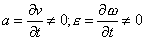(1)

where v – is linear velocity; ω – is angular velocity of a body.

By virtue of an energy – pulse – moment of momentum conservation law the inertia submits to Galilean Law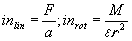(2)

where F – is indirect force applied on a free body; a - linear acceleration of a body; M – is (main) moment of forces applied on a body; ε – is rotary acceleration of rotation of a body around of its center of inertia; inlin – is linear inertia [kg]; inrot – is rotary inertia [kg].

Thus, in the Nature there are two kinds of inertia: linear and rotary. The linear inertia in usual Earth conditions is proportional to weight of material, as the forces of linear inertia Fin and force of gravitation Fgr are proportional to quantity of material

Fin = ma = in∙a; Fgr = mg

The inertia, instead of mass is an accumulator of a momentum and kinetic energy. In case of linear inertia it is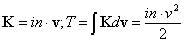where v – is the linear speed of object relatively inertial frame, in which a momentum and kinetic energy of object are measured.

It is understandable that these momentum and energy have relative character, as the value of them will be another in another inertial frame.

We have the other in the case of rotary inertia. It is an accumulator of the moment and rotary kinetic energy invariable at changing of counting frame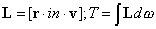While the gravitational field can change vector K for objects having gravitational mass, it is powerless to turn vector L. Therefore vector L of the gyro accumulated energy of rotary inertia, has an invariable direction in the Universe that is in fixed aether. If there would not difference between inertia and gravitation, the gyros, probably, would be turned on a source of gravitational field.

Falsehood of the Principle of Equivalence

For material the measure of linear inertia by virtue of the natural balance of forces having a place in a usual terms, is proportional to a measure of gravitational mass (rest-mass), as in both cases the “manufacturer” of inertia is the same quantity of material.

It is not observed for field. According to experimental data, hypothetical gravitational mass of photon should be no more than 10-20 of its “inertial mass” .

It is not observed for a body, moving with a high velocity. Thus its inertia is various in different directions (“longitudinal” and “transversal" masses) and is not equal to “rest-mass”.

Thus, not only “the principle of equivalence” of gravitational and inertial masses, but also the concept of inertial mass is wrong. That in post - Newtonian physics is named “inertial mass” is not mass but inertia, Galilean measure of interaction of material and aether comprehensively described by Descartes.

The equation, which is as though discovered by Dirac, is actually the usual equation of active (not dependent from velocity) and reactive (velocity dependent) components of inertia .

As it is established still in 1870 - 1874 by Nickolay Umov , the inertia is a development of elastic properties of environment and causality (continuity) of process of energy - momentum transmission, so for aether, carrier environment the following ratio is fair

 dE/dρ = c2. (3)

That is the attitude of density of energy to density of environment or specific inertia is constant and equal to a square of velocity of light.

Naturally, in this case it is density of inertia, instead of density of gravitational mass.

Many experiments (Kozyrev, Podkletnov, Roschin & Godin) have shown that gravitational mass, that is force of interaction of material and gravitational field, is variable in non-equilibrium conditions.

Thus, the false principle of equivalence of Einstein was grounded only on an external likeness of forces of linear inertia and gravitation without deep analysis of reasons causing this phenomenon.

Actual physical world is absolutely another than filled by absolute abstract emptiness abstract - mathematical world of Newton - Poincaré - Hilbert - Einstein - Minkowski. For discover it, we will consider concept of the matter being a key to the given problem.

The Concept of Matter

 “... The killing majority of things, even in cleanly material nature, remain hidden for our pity five or six feelings, and that, hence, the limitation absolutely eliminating these latent participating factors, is limitation really, of a horrifying volume. Such limitation of natural-science pattern of the world only in directly accessible to observation can be termed human that is adapted to a human nature as it in advance builds all on our feelings. But it contradicts as well to a human nature as it is not considered with ability of human spirit and with aspiration proper in him to recreate in the imagination corresponding patterns of the hidden from us participating factors.” Philipp von Lenard, 

Both vulgar idealism of Newtonism, and vulgar materialism of Marxism declaring that “the matter is the physical reality given us in sensation” has reduced the matter to material (substance) having mass and the perceptive forms of physical fields. The positivism, leaving further away from the True, postulated the absence of any that we do not see. Einstein asked: "Do you believe that the moon exists only when you look at it?” .

The relativism declares relativity of the physical phenomenon, its dependence from the process of observation.

Substantially any relativity arises only on the basis of something more close to the Absolute. Material (weighty substance) and field are physical objects, processes and phenomena arising as marine foam on a wave crest in more close to the Absolute aether, main physical matter of the Universe.

In the causal world any existing thing has a reason of existence as in time (in past), and in space (in the constituents). Any composite physical phenomenon, object, process is composition of more simple essences. So the world is divided on more simple less divisible and more uniform initial elements, first causes. If at the macroscopic level each stone is individuality, at the microscopic level there is no property of individuality of units. Each level of complexity of the matter should have simpler base level. For stones a base level is the level of crystalline domains. The level of molecules and atoms is lower it. The level of fundamental particles is lower.

Base level for fundamental particles is by a unrecognized modern relativistic physics the ether.

Within the framework of the concept developed by the author the ether consists of corpuscles (Democritean amers) representing whirligigs, gyros, which can exist in two gyro states - balance (corpuscular, fixed) and unbalanced (phase, existing in persistent motion). Ether has three components, three phase states condition:

- Corpuscular aether (base fixed matter, pseudo-solid);

- Bound phase aether (gravitational aether, amers linking corpuscular aether in domains, therefore aether gains properties of pseudo-liquid);

- Free phase aether (electromagnetic aether, pseudo-gas).

Besides it is possible mark that today the base level for aether, proto-aether only is intended .

The difference of three kinds of aether consists only in the forms of motion of amers and structure of environment. Therefore measures of motion, linear and angular velocity, momentum and moment of momentum, kinetic and potential energy, should be considered and correctly cleared for penetration into essence of the matter.

For deployment of elementary properties of universal matter, aether we will consider its primary indivisible unit, the amer.

Amer, the rotator

Follow to thought of great Kelvin, we present simplistically a corpuscle of aether (Democrites’ amer) as a rotator that is system of rotated inert point and solid inertia less rim or orb or a simple circle having inertial property.

The full mechanical energy of a rotator is determined by a Hamiltonian function H and is equivalent to an internal kinetic energy T of a rotator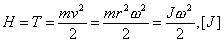(4)

where m – is inertia of a rotated point, v – linear velocity of a point; ω – is angular rate (frequency) of rotation of an inertial point; J – is a moment of inertia.

Generalized momentum h conjugated with angular coordinate, is equal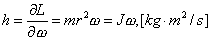(5)

Thus, h is a moment of momentum, and H is possible to express as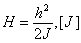On canonical equations of Hamilton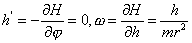(6)

First of equations (6) yields h = M = const, that is conservation law of a moment of momentum. It also is physical sense of Plank constant, instead of invented “action”, which has no physical analogs or physical sense.

The inertial part of amer has insignificant small linear inertia therefore practically it is impossible to measure linear inertia of aether, massif of amers. However rotation rate of this inertial particle is so great, that amer has a final moment of momentum equal to Planck constant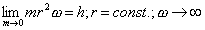(7)

As it is very important problem, let’s consider it in more detail.

Aether, infinite massif of amers

As it is clarified by the author , the ether is infinite in time and space massif of corpuscles, amers, existing in a balance thermodynamic state, only by places and occasionally deviating from the universal balance. Its temperature (the kinetic energy) almost everywhere is close to the triple critical point of aether 2.72°K.

Thus, three levels of aether: corpuscular, bound and free are the prototypes and primogenitors of aggregative states of substance: solid, liquid and gaseous.

Therefore, the models of aether designed in different years by outstanding physicists: gaseous models of aether (Democritus, Descartes, Huygens, Lomonosov, LeSage, Helmholtz, Mendeleyev, A. Nimiriazev , Kasterin , Arp , Atsukovsky  …), liquid models of aether (D. Bernoulli, Euler, Faraday, Maxwell, Kelvin …), solid-state models of aether (McCulagh , Winterberg , Gorbatsevich [24,25] …) despite of large distinction are fair in the aspects and are the basis for future development. Indeed, aether, the Universal matter is infinite and is borderless both in genesis and in the forms of manifestation.

Each amer has own “random” direction of rotation axis that gives a property of amorphism for macroscopic volumes of aether, and therefore isotropy. As in the Universe the causality reigns that is the conservation laws including conservation law of a moment of momentum exists, internal motion of each amer, being practically insulated from an external world, see ω→∞ In (7), has practically identical moment of momentum (5), equal to Planck constant which is not vary at “snail speeds” of substance and field.

The first reason of the Law of Inertia

As against of substance the ether according to (7) practically has no linear inertia and accordingly it has no tractive resistance for motion of substance through it. However its angular inertia, which it has according to Galilean Law (2) is rather great.

The inertial forces in aether arise only at the applying of the moment of forces that it is possible at a close-packed arrangement of aether as it saw in solid models of McCulagh – Winterberg - Gorbatsevich.

Thus,

Aether is “transparent” for rectilinear motions of substance in any direction.

It is one of reasons of Galilean Law of Inertia.

The second reason of the Law of Inertia

In aether of rotators, as well as in any elastic physical environment, there are wave motions. As well as in other environments, it are longitudinal (sound) waves of pressure, in which each cycle the kinetic energy of linear motion of amers environment converts to an internal energy of amers. In connection with an extremely large elasticity of amers this speed also is extremely great . It is possible to consider such waves as almost inertia less.

There are transverse pseudo-shift waves in aether of rotators. Its velocity is known. It is a speed of light (3). Those waves are pseudo-shift because its “shift” is gyroscopic response on applied external moment of forces. Inertial density of free (electromagnetic) ether is 2.8 kg/m3 .

As it was clarified by the author , the substance and field is two forms of poles of oscillations of aether, around of which there are aethereal waves. These associated waves generate linear inertia (momentum) of substance and field, just as the waves in a liquid generate “associated masses” . The modern physics names it as “inertial mass”.

As is established by classic of physics since Galileo and in a final view by N. Umov, any accelerated motion in environment brings about energy - momentum transmission, reconfiguration of field. Thus if environment is linearly-elastic, its inertia does not depend on value of an applied force, and the acceleration is proportional to force. It is the second reason of Galilean Law of Inertia.

Thus,

The Galilean Law of Inertia is an effect of properties of aether, carrier and environment consisting of rotators.

Conclusions

As a result of the study the author has clarified following:

- The outlooks of Newtonian – Einsteinian physics eliminating aether do not correspond to physical reality. It is detected in numerous physical experiments.

- Mass and inertia are different phenomena; the Principle of Equivalence is false.

- The inertia is response of aether to change of motion in it.

- In the Nature there are two forms of inertia - linear and rotary, having different properties.

- Representing aether as highly-packed environment of rotators it is possible to explain reasons of laws of inertia, which appear by an effect of properties of aether.

Acknowledgments

The author expresses the gratitude to all physicists, who supported the present study, in particular to Dr. Felix F. Gorbatsevich (Scientific Centre of Apatite, Russia), whose physically clear and honour researches  have inspired the author on activity in the field of the theory of aether, have yielded an exact base direction of study, to Professor Friedwardt Winterberg (Nevada State University, Reno, USA), the alive classic of nuclear physics and Nickolay Noskov (The National Nuclear Center of Kazakhstan, Almaty, Kazakhstan), whose persistent scientific and intellectual support was a key factor in strengthening of my confidence in a correctness of selected path, to the Dr. Vladimir A. Atsukowski, the first fighter for rehabilitation of aethereal physics and first pathfinder of researches in the field of the theory of aether in modern USSR/Russia who supported study of the author since the first paper , to Dr. Halton C. Arp (Max Planck Astrophysical Institute, Germany), the alive classic of astrophysics, whose support of the aethereal project gave a confidence of a capability of achievement of positive results, to Professor Alexey A. Potapov (The Institute of Dynamics of Systems and Theory of Control of the Siberian Branch of the Russian Academy of Sciences, Irkutsk, Russia), whose remarks and corrections have essentially improved quality of the present study.

Karim Khaidarov,
Almaty, February 15, 2006

References

1. Galileo Galilei – Discorsi e dimostrazioni matematiche intorno a due nuove seinze, attenenti alia meceanica ed ai movimenti locali, 1638.
2. Ньютон И. Математические начала философии, Лондон, 1687.
3. Яворский Б.М., Детлаф А.А. Справочник по физике для инженеров и ст., М., “Наука”, 1968.
4. Декарт Р. Начала философии, Париж, 1644.
5. Ишлинский А.Ю. Механика относительного движения и силы инерции. – М., “Наука”, 1981.
6. Гулиа Н.В. Инерция. – М., “Наука”, 1982.
7. Хайдаров К.А. Эфирная механика. – Боровое, 2004, НиТ, Киев, 2004
8. Хайдаров К.А. Эфир – великий часовщик. – НиТ, Киев, 2004.
9. Хайдаров К.А. Происхождение масс посредством возмущения природного эфира. – SciTecLibrary, 2004.
10. Лебег А. Теория меры. – СПб., 1905.
11. Умов Н.А. Уравнения движения энергии в телах, 1874.
12. Умов Н.А. Добавления к “Уравнениям движения энергии в телах”, 1874
13. Компанеец А. И. Борьба Умова за материализм в физике. – М., 1954.
14. Физический энциклопедический словарь. – М., “СЭ”, 1984.
15. Ленард Ф. Принцип относительности, эфир, гравитация. – М., ГосИз, 1922.
16. Pais A. The Science and Life of Albert Einstein. – Oxford, 1982.
17. Хайдаров К.А. Термодинамика эфира. - Боровое, 2003.
18. Тимирязев А.К. Кинетическая теория материи, М., 1954.
19. Кастерин Н.П. Обобщение основных уравнений аэродинамики и электродинамики. Доклад на особом совещании при Академии наук СССР 9 декабря 1936 г. – М., 1936
20. Arp H. C. The Observational Impetus for Le Saga Gravity. – M-P. Institute fuer Astrophysik, 2001.
21. Ацюковский В.А. Общая эфиродинамика, - М., “Энергоатомиздат”, 1990.
22. MacCullagh J. Brit. Asoc. Rep., 1835
23. Winterberg F. Planck Aether Hypothesis. Karl Gauss Press, Reno, 2002.
24. Горбацевич Ф.Ф. Основы теории непустого эфира. – Апатиты, изд. “Милори”, 1998, 48с.
25. Горбацевич Ф.Ф. Эфирная среда и универсум. – СПб, 2004, 110 с..
26. Хайдаров К.А. Быстрая гравитация, - BRI, Боровое, 2003.
27. Хайдаров К.А. Природа света, - BRI, Боровое, 2004.
28. Риман И.С., Крепс Р.Л. Присоединенные массы тел различной формы. М., 1947.
29. Хайдаров К.А. Вечная Вселенная, - Боровое, НиТ – Киев, 2003.
30. Хайдаров К.А. Эфир: структура и ядерные силы. – BRI, Алматы, 2005.

main page   list of papers   library